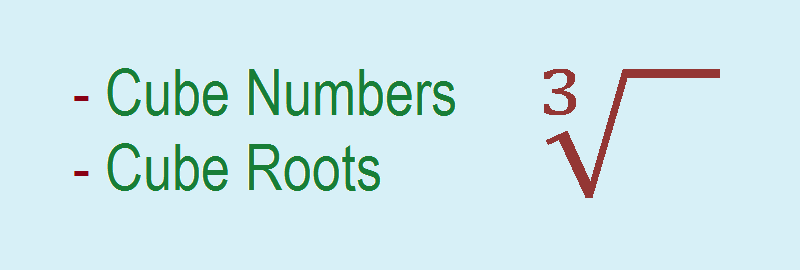# Cube and Cube Roots## Cube and Cube Roots

### Cube Numbers

A number obtained by multiplying any number three times is called a cube number. It is represented by giving the power 3 to the multiplicand.
For example,
Cube of 0 = 03 = 0 × 0 × 0 = 0
Cube of 1 = 13 = 1 × 1 × 1 = 1
Cube of 2 = 23 = 2 × 2 × 2 = 8
Cube of 3 = 33 = 3 × 3 × 3 = 27
Cube of 4 = 43 = 4 × 4 × 4 = 64
Cube of 5 = 53 = 5 × 5 × 5 = 125 and so on.

#### Perfect Cube Numbers

Cube of whole numbers i.e 0, 1, 8, 27, 64, 125, 216, 343, 512, 729, 1000, … etc are called perfect cube numbers.

#### Some facts of cube numbers:

1.    Cube of even positive integer is even.
2.    Cube of positive integer is a positive number.
3.    Cube of negative integer is s negative number.
4.    Cube of odd positive integer is odd.

### Cube Root

Cube number and cube root are correlated. When we multiply three same numbers we get a cube number, and that multiplicand of the cube number is called a cube root. It is represented by giving power 1/3 to the cube number or using radical sign of cube root as shown below:

### Cube root can be calculated by using the prime factorization methods:

In this method we factorize the number into its prime factors and form the power of 3.

For example,

### Workout Examples

Example 1: Find the cube of 11.

Solution: Here,
Cube of 11 = 113 = 11 × 11 × 11 = 1331

Example 2: Find the cube root of 1728 by prime factorization method.

Solution: Here,

You can comment your questions or problems regarding the cube numbers and cube root here.